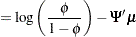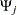Analyses in the LOGISTIC Statement

Likelihood Ratio Chi-Square Test for One Predictor (TEST=LRCHI)

The power computing formula is based on Shieh and O’Brien (1998), Shieh (2000), and Self, Mauritsen, and Ohara (1992).

Define the following notation for a logistic regression analysis: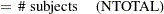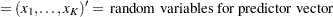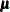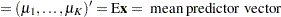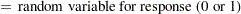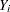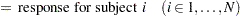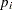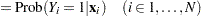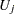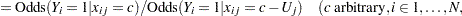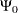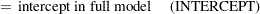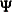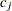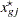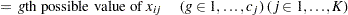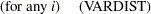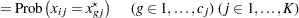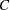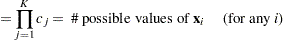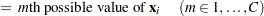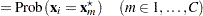The logistic regression model isThe hypothesis test of the first predictor variable is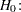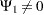Assuming independence among all predictor variables,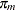is defined as follows:where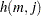is calculated according to the following algorithm: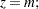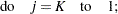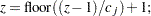This algorithm causes the elements of the transposed vectorto vary fastest to slowest from right to left asincreases, as shown in the following table ofvalues:The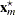values are determined in a completely analogous manner.

The discretization is handled as follows (unless the distribution is ordinal, or binomial with sample size parameter at least as large as requested number of bins): for, generate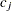quantiles at evenly spaced probability values such that each such quantile is at the midpoint of a bin with probability
. In other words,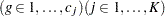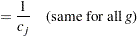The primary noncentrality for the power computation is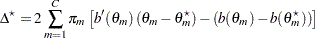where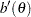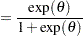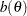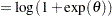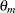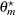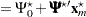where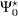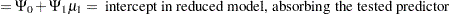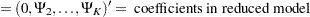The power isAlternative input parameterizations are handled by the following transformations: